# Pre-Algebra : Negative Numbers

## Example Questions

### Example Question #71 : Negative Numbers

Solve: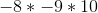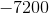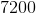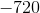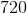Explanation:

When multiplying with more than two integers, we count the number of negative signs. Since there are two, that means our answer is going to be positive. We multiply from left to right. Answer is.

### Example Question #1 : Divide Integers: Ccss.Math.Content.7.Ns.A.2b

Solve: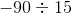Explanation:

When dividing a negative number and a positive number, our answer is negative. Divide normally. Answer is.

### Example Question #1 : Divide Integers: Ccss.Math.Content.7.Ns.A.2b

Solve: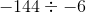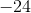Explanation:

When dividing two negative numbers, the answer is positive. Then divide normally. Answer is.

### Example Question #72 : Negative Numbers

Solve: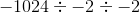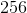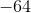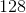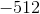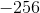Explanation:

When dividing with more than two integers, we count the number of negative signs. Since there are three, that means our answer is going to be negative. We divide from left to right. Answer is.

### Example Question #1 : Add And Subtract Rational Numbers: Ccss.Math.Content.7.Ns.A.1d

Solve.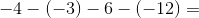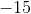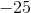Explanation: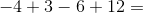### Example Question #71 : Negative Numbers

Solve the following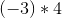Explanation:

Let's refresh our memories about how regular multiplication works.

When you mulitply 2 positive integers (let's say a and b), you are combining groups of a.  For example: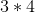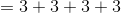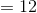You have 4 groups of 3, and you are adding them together to get 12.

We will do the same thing with negative integers.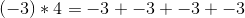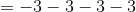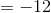So, the solution is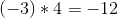### Example Question #291 : Operations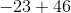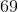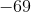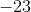Explanation:Since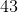is greater thanand is positive, our answer is positive. We treat as a normal subtraction.is the answer.

### Example Question #72 : Negative Numbers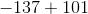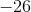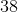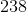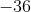Explanation:Since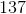is greater than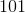and is negative, our answer is negative. We treat as a normal subtraction.

Answer is.

### Example Question #53 : The Number System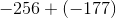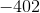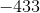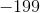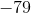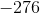Explanation:When adding with another negative number, just treat as an addition problem and then add a negative sign in front.

Answer is### Example Question #71 : Negative Numbers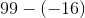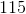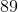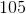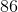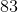Two negative signs become a plus sign. This is now an addition problem.
Answer is